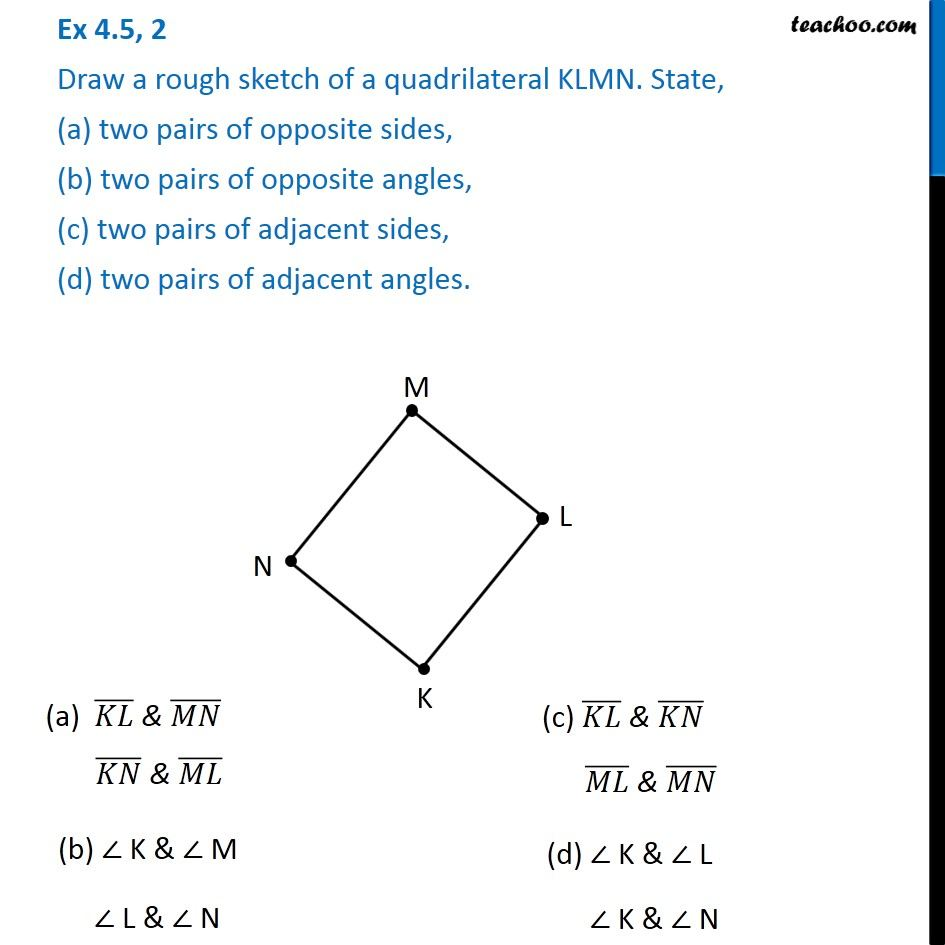1. Chapter 4 Class 6 Basic Geometrical Ideas
2. Serial order wise
3. Ex 4.5

Transcript

Ex 4.5, 2 Draw a rough sketch of a quadrilateral KLMN. State, (a) two pairs of opposite sides, (b) two pairs of opposite angles, (c) two pairs of adjacent sides, (d) two pairs of adjacent angles. . . (a) (𝐾𝐿) ̅ & (𝑀𝑁) ̅ (𝐾𝑁) ̅ & (𝑀𝐿) ̅ (b) ∠ K & ∠ M ∠ L & ∠ N (d) ∠ K & ∠ L ∠ K & ∠ N (c) (𝐾𝐿) ̅ & (𝐾𝑁) ̅ (𝑀𝐿) ̅ & (𝑀𝑁) ̅